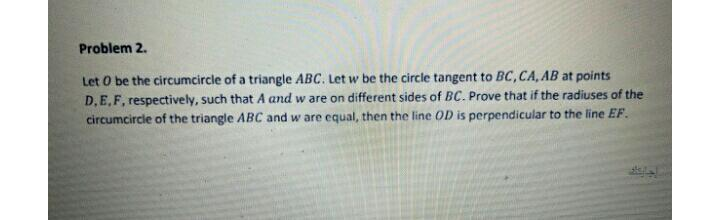Question:

# Problem 2. Let O be the circumcircle of a triangle ABC. Let w be the circle tangent to BC, CA,AB at points D,E,F, respectively,Problem 2. Let O be the circumcircle of a triangle ABC. Let w be the circle tangent to BC, CA,AB at points D,E,F, respectively, such that A and w are on different sides of BC. Prove that if the radiuses of the circumcircle of the triangle ABC and w are equal, then the line OD is perpendicular to the line EF.